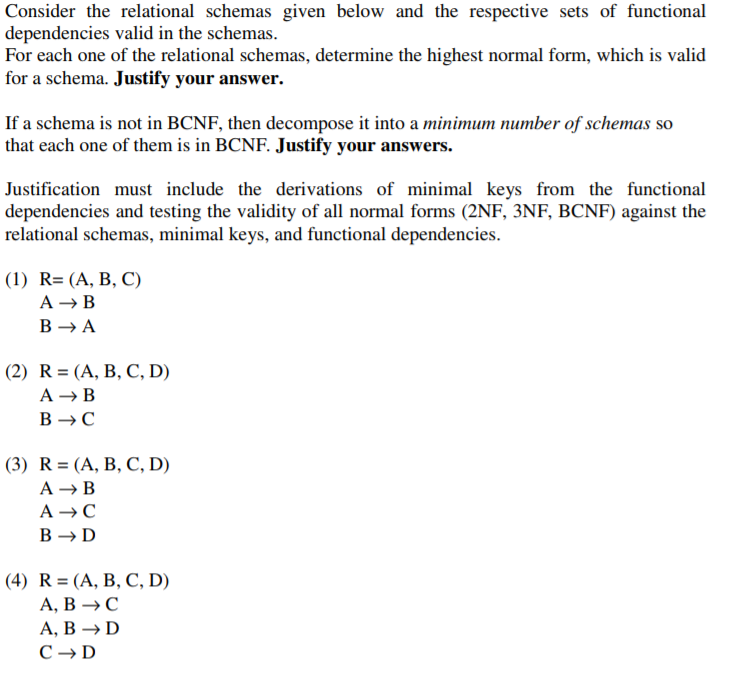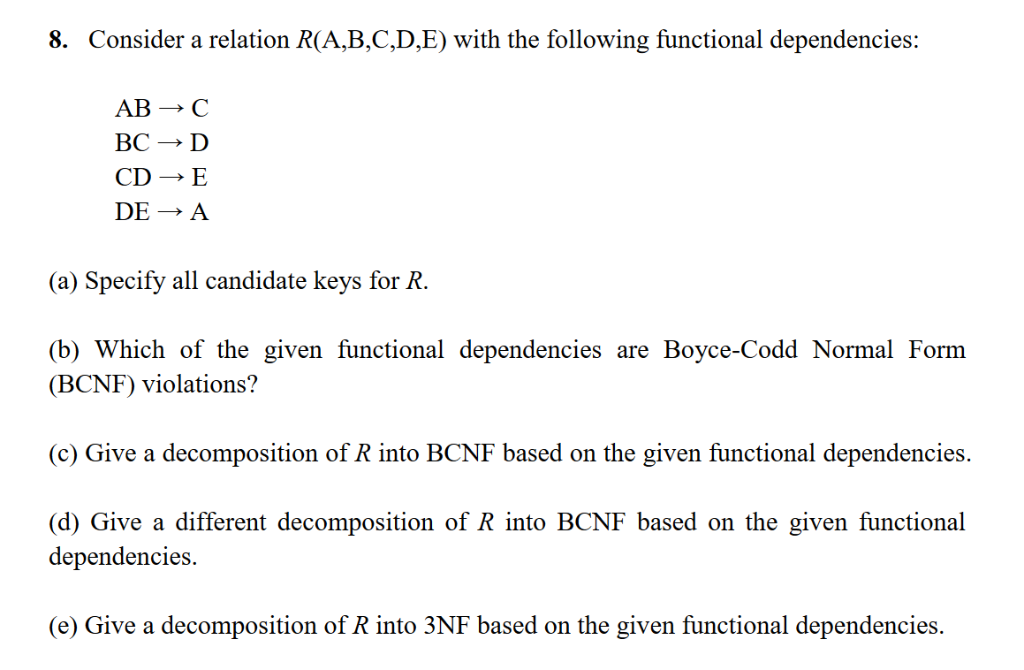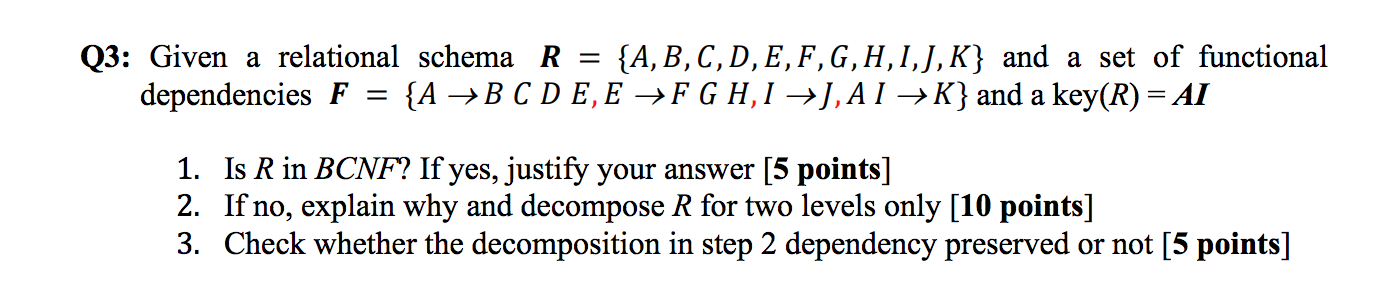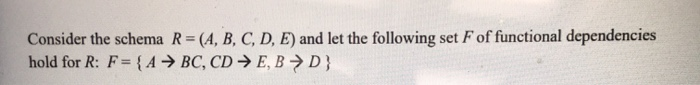Question

# given a set of functional dependencies

given a set of functional dependencies

F = {AB → C, C → B}, above the relational scheme R (A, B, C).

Prove that {AB → AC, AB → BC, AC → B, AC → AB} F +

Solution:

Given,

=>Set of functional dependency F = {AB -> C, C -> B}

=>Relation = R(A, B, C)

To prove: F+ = {AB -> AC, AB -> BC, AC -> B, AC -> AB}

Explanation:

Deriving AB -> AC:

=>(AB)+ = ABC using functional dependency {AB -> C, C -> B} hence we can write AB -> AC

Deriving AB -> BC:

=>(AB)+ = ABC using functional dependency {AB -> C, C -> B} hence we can write AB -> BC

Deriving AC -> B:

=>(AC)+ = ABC using functional dependency {AB -> C, C -> B} hence we can write AC -> B

Deriving AC -> AB:

=>(AC)+ = ABC using functional dependency {AB -> C, C -> B} hence we can write AC -> AB.

=>As we can see that we can cover all the functional dependency of the F+ set using attribute closure; hence F+ set is correct.

=>Hence based on the above statements, we have proved our result.

#### Earn Coins

Coins can be redeemed for fabulous gifts.

Similar Homework Help Questions
• ### Consider the relational schemas given below and the respective sets of functional dependencies va...Write the complete proof. Consider the relational schemas given below and the respective sets of functional dependencies valid in the schemas For each one of the relational schemas, determine the highest normal form, which is valid for a schema. Justify your answer If a schema is not in BCNF, then decompose it into a minimum number of schemas so that each one of them is in BCNF. Justify your answers. Justification must include the derivations of minimal keys from the...

• ### Consider the following definition of equivalent sets of functional dependencies on a relation: “Two sets of...

Consider the following definition of equivalent sets of functional dependencies on a relation: “Two sets of functional dependencies F and F’ on a relation R are equivalent if all FD’s in F’ follow from the ones in F, and all the FD’s in F follow from the ones in F’.” Given a relation R(A, B, C) with the following sets of functional dependencies: F1 = {A  B, B  C}, F2 = {A  B, A  C}, and...

• ### Given a schema R (A, B, C, D, E, F)and a set Fof functional dependencies {A...

Given a schema R (A, B, C, D, E, F)and a set Fof functional dependencies {A →B, A →D, CD →E, CD →F, C →F, C →E, BD →E}, find the closure of the set of functional dependencies ?+

• ### Consider a relation R(A,B,C,D,E) with the following functional dependencies: 8. AB C BCD CDE DEA (a) Specify all candidate keys for R. (b) Which of the given functional dependencies are Boyce-Codd No...Consider a relation R(A,B,C,D,E) with the following functional dependencies: 8. AB C BCD CDE DEA (a) Specify all candidate keys for R. (b) Which of the given functional dependencies are Boyce-Codd Normal Form (BCNF) violations'? (c) Give a decomposition of R into BCNF based on the given functional dependencies. (d) Give a different decomposition of R into BCNF based on the given functional dependencies. (e) Give a decomposition of R into 3NF based on the given functional dependencies. Consider a...

• ### We now have relational scheme R = {A, B, C, D, E, F, G } and the following functional dependencies: D → AC GA → C E → A GD → BF GD → E And the Following MVDs: C→→ A F →→ B GB →→ C Asking: Is this...

We now have relational scheme R = {A, B, C, D, E, F, G } and the following functional dependencies: D → AC GA → C E → A GD → BF GD → E And the Following MVDs: C→→ A F →→ B GB →→ C Asking: Is this relation in the 4NF? Why? If it is not in 4NF, transform it into 4NF.

• ### Q3: Given a relational schema R = {A,B,C,D,E,F,G,H,1,J,K} and a set of functional dependencies F {A...Q3: Given a relational schema R = {A,B,C,D,E,F,G,H,1,J,K} and a set of functional dependencies F {A B C D E, E F G H I J,AI →K} and a key(R) = AI = 1. Is R in BCNF? If yes, justify your answer [5 points] 2. If no, explain why and decompose R for two levels only [10 points] 3. Check whether the decomposition in step 2 dependency preserved or not [5 points]

• ### 1. Consider the relation PatientOf and the functional dependencies below. Describe, with examples, how redundancy, update,...

1. Consider the relation PatientOf and the functional dependencies below. Describe, with examples, how redundancy, update, and delete anomalies can arise. PatientOf (patient_no, name, address, doctor_no, since) patient_no → name, address patient_no, doctor_no → since 2. Consider a relation with attributes R(A, B, C, D, E) that satisfies the following functional dependencies: AB → D AC → E BC → D D → A E → B Find all the keys that contain the attribute A.

• ### Here's a relation (R), its attributes and its functional dependencies (F): R(A, B, C, D, E)...

Here's a relation (R), its attributes and its functional dependencies (F): R(A, B, C, D, E) C D → B A → D D → C E → C What is the closure of AB ({AB}+)? What is the closure of F (F+)? [ set of closures for all LHS][each LHS on one line] What is the minimal set (cover) for F? Provide a key for relation R (a minimal set of attributes that can determine all attr.) Decompose the...

• ### Consider the schema R=(A, B, C, D, E) and let the following set F of functional...Consider the schema R=(A, B, C, D, E) and let the following set F of functional dependencies hold for R: F= {A → BC, CD → E, B D } Problem 3 Suppose that the schema R=(A, B, C, D, E) is decomposed into R/ - (A, B, C) and R=(A, D, E). Show if this decomposition is a lossless decomposition with respect to the given set of functional dependencies F.

• ### Consider a relation R(A, B, C, D) with the functional dependencies {AB → C, C →...

Consider a relation R(A, B, C, D) with the functional dependencies {AB → C, C → D, D → A}. Does BC → A hold on R? Explain.    show steps.